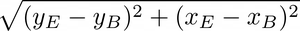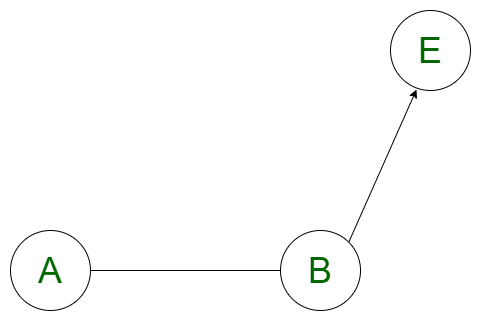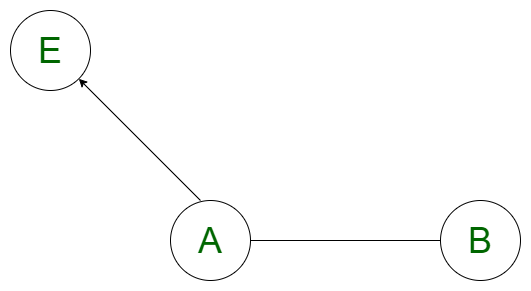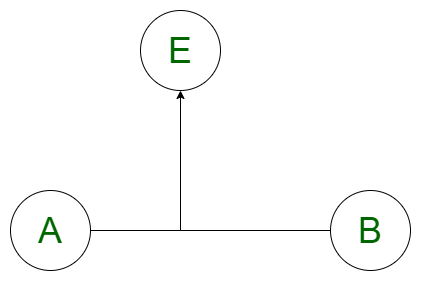# Minimum distance from a point to the line segment using Vectors

• Difficulty Level : Easy
• Last Updated : 01 Jun, 2021

Given the coordinates of two endpoints A(x1, y1), B(x2, y2) of the line segment and coordinates of a point E(x, y); the task is to find the minimum distance from the point to line segment formed with the given coordinates.
Note that both the ends of a line can go to infinity i.e. a line has no ending points. On the other hand, a line segment has start and endpoints due to which length of the line segment is fixed.
Examples:

Input: A = {0, 0}, B = {2, 0}, E = {4, 0}

Attention reader! All those who say programming isn't for kids, just haven't met the right mentors yet. Join the  Demo Class for First Step to Coding Coursespecifically designed for students of class 8 to 12.

The students will get to learn more about the world of programming in these free classes which will definitely help them in making a wise career choice in the future.

Output:
To find the distance, dot product has to be found between vectors AB, BE and AB, AE.
AB = (x2 – x1, y2 – y1) = (2 – 0, 0 – 0) = (2, 0)
BE = (x – x2, y – y2) = (4 – 2, 0 – 0) = (2, 0)
AE = (x – x1, y – y1) = (4 – 0, 0 – 0) = (4, 0)
AB . BE = (ABx * BEx + ABy * BEy) = (2 * 2 + 0 * 0) = 4
AB . AE = (ABx * AEx + ABy * AEy) = (2 * 4 + 0 * 0) = 8
Therefore, nearest point from E to line segment is point B.
Minimum Distance = BE == 2
Input: A = {0, 0}, B = {2, 0}, E = {1, 1}
Output:

Approach: The idea is to use the concept of vectors to solve the problem since the nearest point always lies on the line segment. Assuming that the direction of vector AB is A to B, there are three cases that arise:

1. The nearest point from the point E on the line segment AB is point B itself if the dot product of vector AB(A to B) and vector BE(B to E) is positive where E is the given point. Since AB . BE > 0, the given point lies in the same direction as the vector AB is and the nearest point must be B itself because the nearest point lies on the line segment.2. The nearest point from the point E on the line segment AB is point A itself if the dot product of vector AB(A to B) and vector BE(B to E) is negative where E is the given point. Since AB . BE < 0, the given point lies in the opposite direction of the line segment AB and the nearest point must be A itself because the nearest point lies on the line segment.3. If the dot product is 0, then the point E is perpendicular to the line segment AB and the perpendicular distance to the given point E from the line segment AB is the shortest distance. If some arbitrary point F is the point on the line segment which is perpendicular to E, then the perpendicular distance can be calculated as |EF| = |(AB X AE)/|AB||Below is the implementation of the above approach:

## C++

 `// C++ implementation of the approach``#include ` `// To store the point``#define Point pair``#define F first``#define S second``using` `namespace` `std;` `// Function to return the minimum distance``// between a line segment AB and a point E``double` `minDistance(Point A, Point B, Point E)``{` `    ``// vector AB``    ``pair<``double``, ``double``> AB;``    ``AB.F = B.F - A.F;``    ``AB.S = B.S - A.S;` `    ``// vector BP``    ``pair<``double``, ``double``> BE;``    ``BE.F = E.F - B.F;``    ``BE.S = E.S - B.S;` `    ``// vector AP``    ``pair<``double``, ``double``> AE;``    ``AE.F = E.F - A.F,``    ``AE.S = E.S - A.S;` `    ``// Variables to store dot product``    ``double` `AB_BE, AB_AE;` `    ``// Calculating the dot product``    ``AB_BE = (AB.F * BE.F + AB.S * BE.S);``    ``AB_AE = (AB.F * AE.F + AB.S * AE.S);` `    ``// Minimum distance from``    ``// point E to the line segment``    ``double` `reqAns = 0;` `    ``// Case 1``    ``if` `(AB_BE > 0) {` `        ``// Finding the magnitude``        ``double` `y = E.S - B.S;``        ``double` `x = E.F - B.F;``        ``reqAns = ``sqrt``(x * x + y * y);``    ``}` `    ``// Case 2``    ``else` `if` `(AB_AE < 0) {``        ``double` `y = E.S - A.S;``        ``double` `x = E.F - A.F;``        ``reqAns = ``sqrt``(x * x + y * y);``    ``}` `    ``// Case 3``    ``else` `{` `        ``// Finding the perpendicular distance``        ``double` `x1 = AB.F;``        ``double` `y1 = AB.S;``        ``double` `x2 = AE.F;``        ``double` `y2 = AE.S;``        ``double` `mod = ``sqrt``(x1 * x1 + y1 * y1);``        ``reqAns = ``abs``(x1 * y2 - y1 * x2) / mod;``    ``}``    ``return` `reqAns;``}` `// Driver code``int` `main()``{``    ``Point A = make_pair(0, 0);``    ``Point B = make_pair(2, 0);``    ``Point E = make_pair(1, 1);` `    ``cout << minDistance(A, B, E);` `    ``return` `0;``}`

## Java

 `// Java implementation of the approach``class` `GFG``{` `static` `class` `pair``{``    ``double` `F, S;``    ``public` `pair(``double` `F, ``double` `S)``    ``{``        ``this``.F = F;``        ``this``.S = S;``    ``}``    ``public` `pair() {``    ``}``}` `// Function to return the minimum distance``// between a line segment AB and a point E``static` `double` `minDistance(pair A, pair B, pair E)``{` `    ``// vector AB``    ``pair AB = ``new` `pair();``    ``AB.F = B.F - A.F;``    ``AB.S = B.S - A.S;` `    ``// vector BP``    ``pair BE = ``new` `pair();``    ``BE.F = E.F - B.F;``    ``BE.S = E.S - B.S;` `    ``// vector AP``    ``pair AE = ``new` `pair();``    ``AE.F = E.F - A.F;``    ``AE.S = E.S - A.S;` `    ``// Variables to store dot product``    ``double` `AB_BE, AB_AE;` `    ``// Calculating the dot product``    ``AB_BE = (AB.F * BE.F + AB.S * BE.S);``    ``AB_AE = (AB.F * AE.F + AB.S * AE.S);` `    ``// Minimum distance from``    ``// point E to the line segment``    ``double` `reqAns = ``0``;` `    ``// Case 1``    ``if` `(AB_BE > ``0``)``    ``{` `        ``// Finding the magnitude``        ``double` `y = E.S - B.S;``        ``double` `x = E.F - B.F;``        ``reqAns = Math.sqrt(x * x + y * y);``    ``}` `    ``// Case 2``    ``else` `if` `(AB_AE < ``0``)``    ``{``        ``double` `y = E.S - A.S;``        ``double` `x = E.F - A.F;``        ``reqAns = Math.sqrt(x * x + y * y);``    ``}` `    ``// Case 3``    ``else``    ``{` `        ``// Finding the perpendicular distance``        ``double` `x1 = AB.F;``        ``double` `y1 = AB.S;``        ``double` `x2 = AE.F;``        ``double` `y2 = AE.S;``        ``double` `mod = Math.sqrt(x1 * x1 + y1 * y1);``        ``reqAns = Math.abs(x1 * y2 - y1 * x2) / mod;``    ``}``    ``return` `reqAns;``}` `// Driver code``public` `static` `void` `main(String[] args)``{``    ``pair A = ``new` `pair(``0``, ``0``);``    ``pair B = ``new` `pair(``2``, ``0``);``    ``pair E = ``new` `pair(``1``, ``1``);` `    ``System.out.print((``int``)minDistance(A, B, E));``}``}` `// This code is contributed by 29AjayKumar`

## Python3

 `# Python3 implementation of the approach``from` `math ``import` `sqrt` `# Function to return the minimum distance``# between a line segment AB and a point E``def` `minDistance(A, B, E) :` `    ``# vector AB``    ``AB ``=` `[``None``, ``None``];``    ``AB[``0``] ``=` `B[``0``] ``-` `A[``0``];``    ``AB[``1``] ``=` `B[``1``] ``-` `A[``1``];` `    ``# vector BP``    ``BE ``=` `[``None``, ``None``];``    ``BE[``0``] ``=` `E[``0``] ``-` `B[``0``];``    ``BE[``1``] ``=` `E[``1``] ``-` `B[``1``];` `    ``# vector AP``    ``AE ``=` `[``None``, ``None``];``    ``AE[``0``] ``=` `E[``0``] ``-` `A[``0``];``    ``AE[``1``] ``=` `E[``1``] ``-` `A[``1``];` `    ``# Variables to store dot product` `    ``# Calculating the dot product``    ``AB_BE ``=` `AB[``0``] ``*` `BE[``0``] ``+` `AB[``1``] ``*` `BE[``1``];``    ``AB_AE ``=` `AB[``0``] ``*` `AE[``0``] ``+` `AB[``1``] ``*` `AE[``1``];` `    ``# Minimum distance from``    ``# point E to the line segment``    ``reqAns ``=` `0``;` `    ``# Case 1``    ``if` `(AB_BE > ``0``) :` `        ``# Finding the magnitude``        ``y ``=` `E[``1``] ``-` `B[``1``];``        ``x ``=` `E[``0``] ``-` `B[``0``];``        ``reqAns ``=` `sqrt(x ``*` `x ``+` `y ``*` `y);` `    ``# Case 2``    ``elif` `(AB_AE < ``0``) :``        ``y ``=` `E[``1``] ``-` `A[``1``];``        ``x ``=` `E[``0``] ``-` `A[``0``];``        ``reqAns ``=` `sqrt(x ``*` `x ``+` `y ``*` `y);` `    ``# Case 3``    ``else``:` `        ``# Finding the perpendicular distance``        ``x1 ``=` `AB[``0``];``        ``y1 ``=` `AB[``1``];``        ``x2 ``=` `AE[``0``];``        ``y2 ``=` `AE[``1``];``        ``mod ``=` `sqrt(x1 ``*` `x1 ``+` `y1 ``*` `y1);``        ``reqAns ``=` `abs``(x1 ``*` `y2 ``-` `y1 ``*` `x2) ``/` `mod;``    ` `    ``return` `reqAns;` `# Driver code``if` `__name__ ``=``=` `"__main__"` `:` `    ``A ``=` `[``0``, ``0``];``    ``B ``=` `[``2``, ``0``];``    ``E ``=` `[``1``, ``1``];` `    ``print``(minDistance(A, B, E));` `# This code is contributed by AnkitRai01`

## C#

 `// C# implementation of the approach``using` `System;` `class` `GFG``{` `class` `pair``{``    ``public` `double` `F, S;``    ``public` `pair(``double` `F, ``double` `S)``    ``{``        ``this``.F = F;``        ``this``.S = S;``    ``}``    ``public` `pair() {``    ``}``}` `// Function to return the minimum distance``// between a line segment AB and a point E``static` `double` `minDistance(pair A, pair B, pair E)``{` `    ``// vector AB``    ``pair AB = ``new` `pair();``    ``AB.F = B.F - A.F;``    ``AB.S = B.S - A.S;` `    ``// vector BP``    ``pair BE = ``new` `pair();``    ``BE.F = E.F - B.F;``    ``BE.S = E.S - B.S;` `    ``// vector AP``    ``pair AE = ``new` `pair();``    ``AE.F = E.F - A.F;``    ``AE.S = E.S - A.S;` `    ``// Variables to store dot product``    ``double` `AB_BE, AB_AE;` `    ``// Calculating the dot product``    ``AB_BE = (AB.F * BE.F + AB.S * BE.S);``    ``AB_AE = (AB.F * AE.F + AB.S * AE.S);` `    ``// Minimum distance from``    ``// point E to the line segment``    ``double` `reqAns = 0;` `    ``// Case 1``    ``if` `(AB_BE > 0)``    ``{` `        ``// Finding the magnitude``        ``double` `y = E.S - B.S;``        ``double` `x = E.F - B.F;``        ``reqAns = Math.Sqrt(x * x + y * y);``    ``}` `    ``// Case 2``    ``else` `if` `(AB_AE < 0)``    ``{``        ``double` `y = E.S - A.S;``        ``double` `x = E.F - A.F;``        ``reqAns = Math.Sqrt(x * x + y * y);``    ``}` `    ``// Case 3``    ``else``    ``{` `        ``// Finding the perpendicular distance``        ``double` `x1 = AB.F;``        ``double` `y1 = AB.S;``        ``double` `x2 = AE.F;``        ``double` `y2 = AE.S;``        ``double` `mod = Math.Sqrt(x1 * x1 + y1 * y1);``        ``reqAns = Math.Abs(x1 * y2 - y1 * x2) / mod;``    ``}``    ``return` `reqAns;``}` `// Driver code``public` `static` `void` `Main(String[] args)``{``    ``pair A = ``new` `pair(0, 0);``    ``pair B = ``new` `pair(2, 0);``    ``pair E = ``new` `pair(1, 1);` `    ``Console.Write((``int``)minDistance(A, B, E));``}``}` `// This code is contributed by 29AjayKumar`

## Javascript

 ``
Output:
`1`

Time Complexity: O(1 )

Auxiliary Space: O(1)

My Personal Notes arrow_drop_up